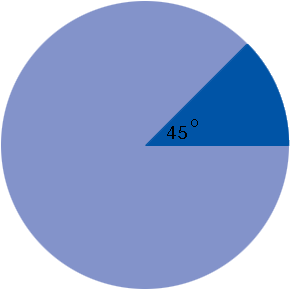SEARCH HOMEMath Central Quandaries & QueriesQuestion from angelkem, a student: how do you get the area of the circle, the sector, the shaded sector, non shaded sector and its total area if the diameter is 20 cm and each area of the circle is 45 degrees?Angelkem,

The area of a circle is π r2 where r is the radius which in your case is 20/2 = 10 cm.There are 360o in the entire circle. 360/45 = 8 so a sector with a central angle of 45o will have an area which is one-eighth the area of the circle.

PennyMath Central is supported by the University of Regina and The Pacific Institute for the Mathematical Sciences.